# 如何在代码层面提高CPU分支预测效率

### 将最常见的条件比较单独从switch中移出

• 直接跳转(PC-relative, direct) ： offset以立即数形式固定在指令中，所以目标地址也是固定的。
• 间接跳转(absolute, indirect)：目标地址来自通用寄存器，而寄存器的值不固定。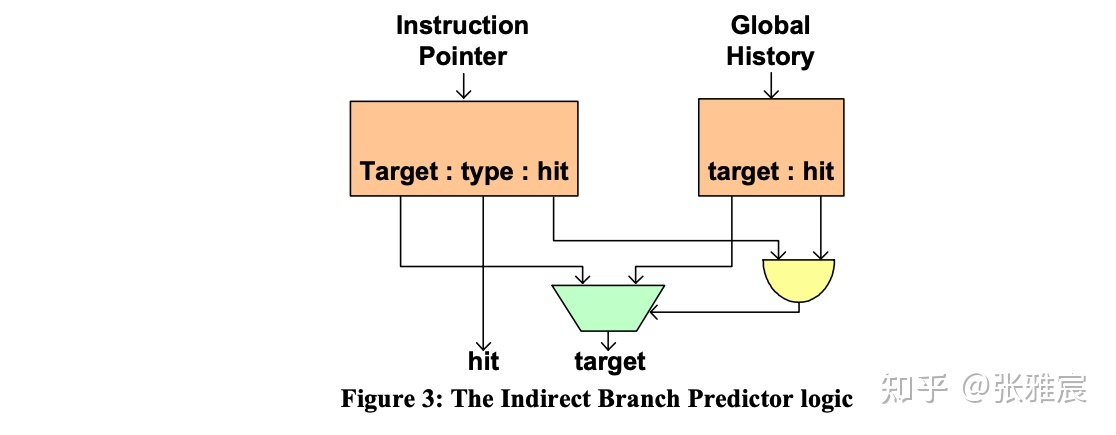switch-case的优点是将诸多if/else(conditional branch)转换为统一的unconditioal branch，但缺点就是目标地址难以预测。如果某个case的命中率特别高，就可以将其从switch中单独提出来，这样该分支的预测方向 && 目标地址都很好预测。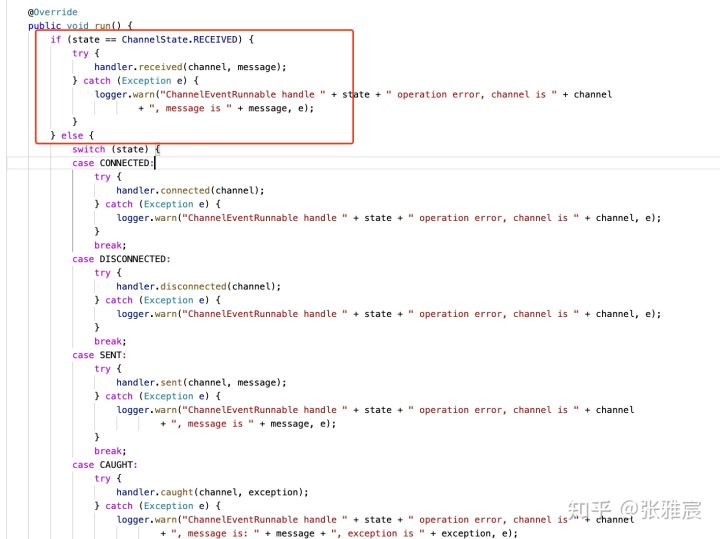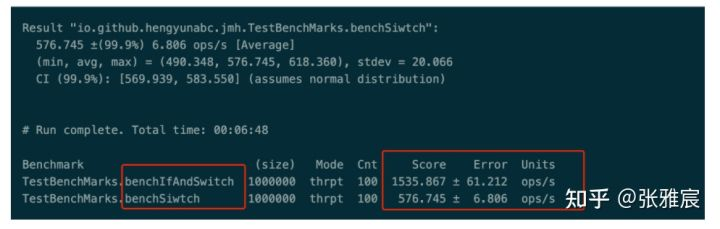### 将使用【控制】的条件转移转换为使用【数据】的条件转移

CMOV指令就是典型的例子。CPU无需进行分支预测，但是会计算一个条件的两种结果，然后通过检查条件码，要么更新目的寄存器，要么保持不变。

``v = test-expr ? then-expr : else-expr``

``v = then-expr; ve = else-expr; t = test-expr; if(!t) v=ve;``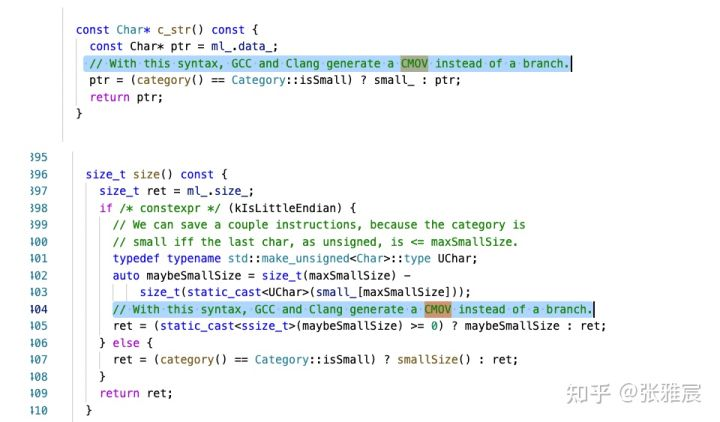``/* Rearrange two vectors so that for each i, b[i] >= a[i] */ void minmax1(long a[], long b[], long n) {   long i;   for (i = 0; i < n; i++) {     if (a[i] > b[i]) {       long t = a[i];       a[i] = b[i];       b[i] = t;     }   } }  /* Rearrange two vectors so that for each i, b[i] >= a[i] */ void minmax2(long a[], long b[], long n) {   long i;   for (i = 0; i < n; i++) {     long min = a[i] < b[i] ? a[i] : b[i];     long max = a[i] < b[i] ? b[i] : a[i];     a[i] = min;     b[i] = max;   } }``

### 使用算数逻辑代替分支

``// origin version int insideRange1(int v, int min, int max) {   return v >= min && v < max; }  // optimized version int insideRange2(int v, int min, int max) {   return (unsigned) (v - min) < (max - min); }``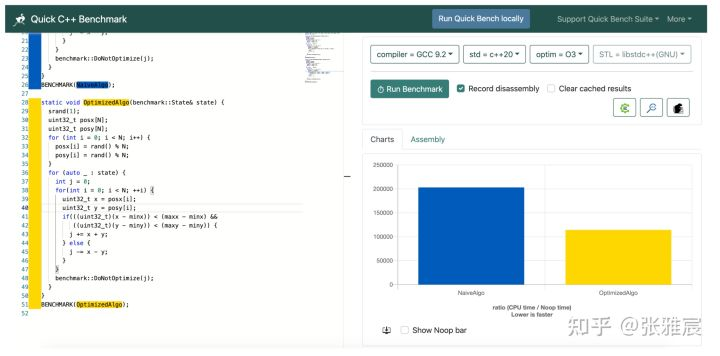Avoiding Branches里有更多的例子，不过用之前还是做测试更靠谱。

### 使用template移除分支

2018年Stephen Yang的博士论文NanoLog: A Nanosecond Scale Logging System介绍了一款C++日志库Nanolog，将日志调用开销的中位数降为了个位数纳秒级别。作者在文章NANOLOG: A NANOSECOND SCALE LOGGING SYSTEM中提到了Nanolog的关键技术和优化，第三条就是将printf在运行时的大量分支逻辑利用C++ template优化成编译期的运算。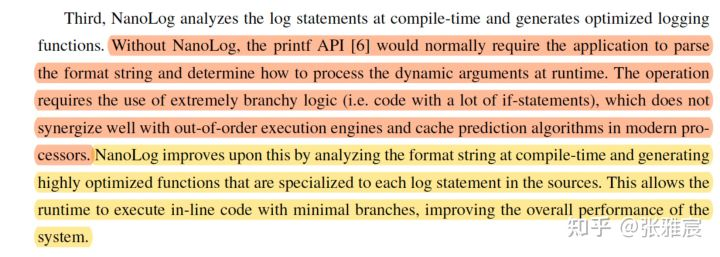### likely/unlikely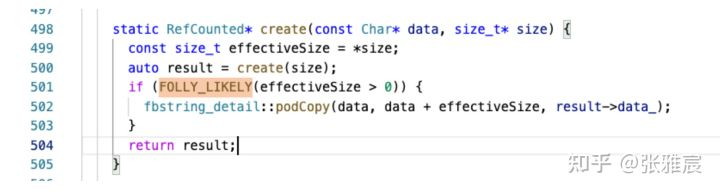`FOLLY_LIKELY`是一个包装：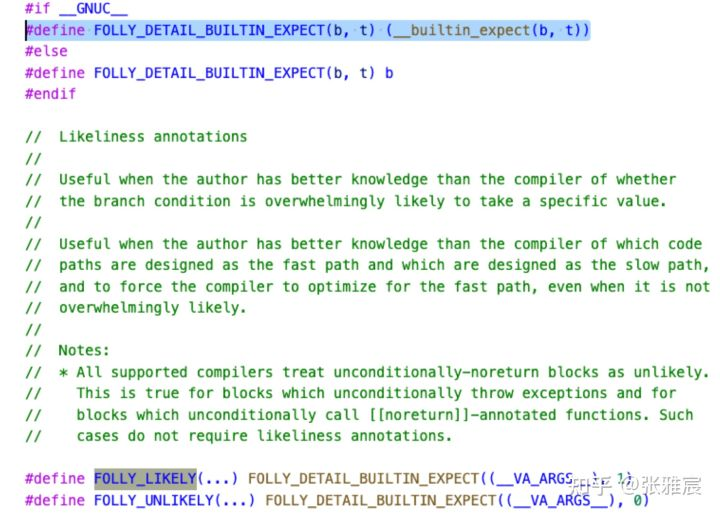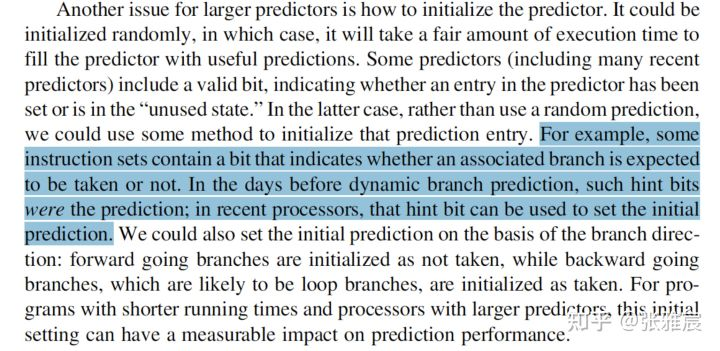(完)